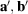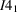International Tables for Crystallography (2016). Vol. A, ch. 1.3, pp. 22-41https://doi.org/10.1107/97809553602060000921

Contents

• 1.3. A general introduction to space groups  (pp. 22-41)
• 1.3.1. Introduction  (p. 22) | html | pdf |
• 1.3.2. Lattices  (pp. 22-28) | html | pdf |
• 1.3.2.1. Basic properties of lattices  (pp. 22-23) | html | pdf |
• 1.3.2.2. Metric properties  (pp. 23-24) | html | pdf |
• 1.3.2.3. Unit cells  (p. 24) | html | pdf |
• 1.3.2.4. Primitive and centred lattices  (pp. 24-27) | html | pdf |
• 1.3.2.5. Reciprocal lattice  (pp. 27-28) | html | pdf |
• 1.3.3. The structure of space groups  (pp. 28-31) | html | pdf |
• 1.3.3.1. Point groups of space groups  (pp. 28-29) | html | pdf |
• 1.3.3.2. Coset decomposition with respect to the translation subgroup  (pp. 29-31) | html | pdf |
• 1.3.3.3. Symmorphic and non-symmorphic space groups  (p. 31) | html | pdf |
• 1.3.4. Classification of space groups  (pp. 31-41) | html | pdf |
• 1.3.4.1. Space-group types  (pp. 31-33) | html | pdf |
• 1.3.4.2. Geometric crystal classes  (pp. 33-34) | html | pdf |
• 1.3.4.3. Bravais types of lattices and Bravais classes  (pp. 34-37) | html | pdf |
• 1.3.4.4. Other classifications of space groups  (pp. 37-41) | html | pdf |
• 1.3.4.4.1. Arithmetic crystal classes  (pp. 37-39) | html | pdf |
• 1.3.4.4.2. Lattice systems  (p. 39) | html | pdf |
• 1.3.4.4.3. Crystal systems  (pp. 39-40) | html | pdf |
• 1.3.4.4.4. Crystal families  (pp. 40-41) | html | pdf |
• References | html | pdf |
• Figures
• Fig. 1.3.2.1. Conventional basisand a non-conventional basisfor the square lattice  (p. 23) | html | pdf |
• Fig. 1.3.2.2. Voronoï domains and primitive unit cells for a rectangular lattice (a) and an oblique lattice (b)  (p. 24) | html | pdf |
• Fig. 1.3.2.3. Primitive rectangular lattice (only the filled nodes) and centred rectangular lattice (filled and open nodes)  (p. 25) | html | pdf |
• Fig. 1.3.2.4. Primitive cell (dashed line) and centred cell (solid lines) for the centred rectangular lattice  (p. 25) | html | pdf |
• Fig. 1.3.4.1. Classification levels for three-dimensional space groups  (p. 32) | html | pdf |
• Fig. 1.3.4.2. Space-group diagram of(left) and its reflection in the plane z = 0 (right)  (p. 33) | html | pdf |
• Tables
• Table 1.3.3.1. Automorphism groups of two-dimensional primitive lattices  (p. 29) | html | pdf |
• Table 1.3.3.2. Automorphism groups of three-dimensional primitive lattices  (p. 30) | html | pdf |
• Table 1.3.3.3. Right-coset decomposition ofrelative to(p. 30) | html | pdf |
• Table 1.3.4.1. Lattice systems in three-dimensional space  (p. 39) | html | pdf |
• Table 1.3.4.2. Crystal systems in three-dimensional space  (p. 39) | html | pdf |
• Table 1.3.4.3. Distribution of space-group types in the hexagonal crystal family  (p. 40) | html | pdf |